TitleCollege Algebra
Answer/Discussion to Practice Problems
Tutorial 39: Zeros of Polynomial Functions, Part II:
Upper and Lower Bounds, Intermediate Value Theorem, Fundamental Theorem of Algebra,
and the Linear Factorization TheoremAnswer/Discussion to 1aIn other words, we need to show that -3 is a lower bound and 4 is an upper bound for real roots of the given equation. Checking the Lower Bound: Lets apply synthetic division with -3 and see if we get alternating signs:Note how c = -3 < 0 AND the successive signs in the bottom row of our synthetic division alternate.  You know what that means? -3 is a lower bound for the real roots of this equation.

 Checking the Upper Bound: Lets apply synthetic division with 4 and see if we get all positive:Note how c = 4 > 0 AND the all of the signs in the bottom row of our synthetic division are positive.  You know what that means? 4 is an upper bound for the real roots of this equation.   Since -3 is a lower bound and 4 is an upper bound for the real roots of the equation, then that means all real roots of the equation lie between -3 and 4.Answer/Discussion to 2aWhen finding functional values, you can either use synthetic division or directly plug the number into the function.  Since we will only be interested in knowing the functional value in this problem, I'm going to directly plug my x value into the function.  If I were needing more, for example the signs of the quotient, like above, then I would use synthetic division.  Finding f(-2):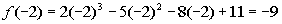Finding f(-1):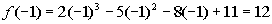Since there is a sign change between f(-2) = -9 and f(-1) = 12, then according to the Intermediate Value Theorem, there is at least one value between -2 and -1 that is a zero of this polynomial function.   Checking functional values at intervals of one-tenth for a sign change:Finding f(-1.1):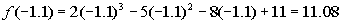Finding f(-1.2):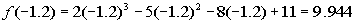Finding f(-1.3):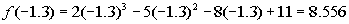Finding f(-1.4):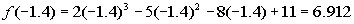Finding f(-1.5):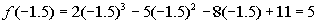Finding f(-1.6):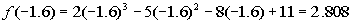Finding f(-1.7):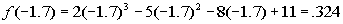Finding f(-1.8):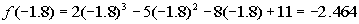Hey we have a sign change!!!!! Now we want to find the zero to the nearest tenth.  So is it going to be x = -1.7 or x = -1.8.  We can not necessarily go by which functional value is closer to zero.  We will need to dig a little bit deeper and go by intervals of one-hundredths:Finding f(-1.71):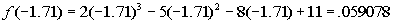Finding f(-1.72):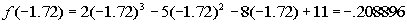Whew!!!!  At last we come to a sign change between successive hundredths.  That means we have it narrowed down at little bit better.  There is a zero between -1.71 and -1.72.  Since it would land slightly below -1.71, the nearest tenth would be -1.7. The work here is not hard, it is just tedious.Answer/Discussion to 3a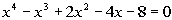Since the complex number 2i is a root, that means it's conjugate -2i is also a root.  This will help us break down the function to find any other roots. This is done in the same fashion as when you are given a real zero. If you need a review on finding roots of a polynomial equation f(x) = 0 when given a root, feel free to go to Tutorial 37: Synthetic Division and the Remainder and Factor Theorems. Using synthetic division to find the quotient we get:Yuck!!!   Look at all of those complex numbers in the quotient.  Don't fear, when we put in our conjugate using that quotient those complex numbers will disappear and we will be left with a nice quotient with real number coefficients. Check it out:Now we are getting somewhere.  From here we can rewrite the original problem using the roots that we have above and the quotient that we ended up with in this last synthetic division.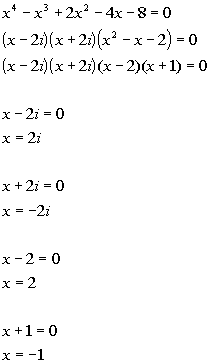*The 1st two factors are x minus the complex zeros *The 3rd factor is the quotient found directly above *Factor the trinomial *The given complex zero     *The conjugate zero     *Setting the 3rd factor = 0   *Setting the 4th factor = 0

 So the roots of the polynomial equation are 2i,  -2i, 2, and -1.Answer/Discussion to 4a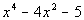Factor as the product of factors that are irreducible over rational numbers: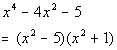*Factor the trinomial

 Since 5 is not a perfect square, this is as far as we can factor it using only rational numbers.   Factor as the product of factors that are irreducible over real numbers: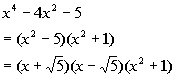*Factor the difference of squares

 Did you know that the sum of squares can be factored over the complex non-real numbers as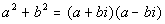? Completely factored form involving complex non-real numbers: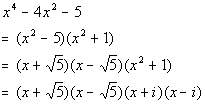Note how once we had factored over the complex numbers that we ended up with four linear factors and that our polynomial was degree four.Answer/Discussion to 5a n = 4; 2, -1, and 2i are zeros; f(1) = -30.

 Step 1: Use the given zeros and the Linear Factorization Theorem to write out all of the factors of the polynomial function.

 Since our degree is 4, that means there are four linear factors over the complex numbers (possibly real and not necessarily distinct). Note how we are only given two zeros.  We need to come up with a third one.  Do you have any ideas? Oh yeah, if a non-real complex number is a zero than its conjugate is also a zero.  Since 2i is a zero, that means -2i is also a zero. Using the Linear Factorization Theorem we get: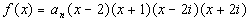Step 2: Multiply all of the factors found in Step 1.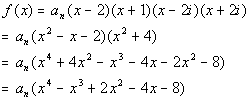*Multiply comp. factors *Multiply remaining factors

 This problem gave another condition, f(1) = -30. This will help us find in this problem.*f(1) = -30 *Solve for a sub n

 Putting it all together we get: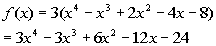Last revised on March 15, 2010 by Kim Seward.
All contents copyright (C) 2002 - 2012, WTAMU and Kim Seward. All rights reserved.